Function Repository Resource:

# TuckerDecomposition

Compute the Tucker decomposition of a tensor

Contributed by: Nikolay Murzin
 ResourceFunction["TuckerDecomposition"][tensor] computes the Tucker decomposition of a given input tensor, returning the core tensor and a list of factor matrices. ResourceFunction["TuckerDecomposition"][tensor,rank] uses a rank specification that determines the truncation level for each dimension (mode, axis) of a tensor.

## Details and Options

The Tucker decomposition is also known as the higher-order singular value decomposition (HOSVD).
The Tucker decomposition expresses a tensor as the multilinear product of a "core tensor" and a set of unitary factor matrices.
ResourceFunction["TuckerDecomposition"] computes the Tucker decomposition of an input tensor by iteratively applying the singular value decomposition (SVD) to a series of matrices obtained by flattening a tensor along its dimensions.
ResourceFunction["TuckerDecomposition"] internally computes an effective precision for a tensor and ensures that it has a depth of at least 2. If a tensor depth is less than 2, it returns an input tensor as a core and an empty list of factors.
rank can be specified as a list or as a single value, which is then expanded to a list with the same length as an input tensor's depth. If a rank is set to Infinity, the function will not truncate any singular values.
ResourceFunction["TuckerDecomposition"] accepts the same options as SingularValueDecomposition.
The Tolerance option is used to truncate singular values across each dimension of the tensor.
The function iteratively applies SVD to a tensor, keeping singular vectors corresponding to non-zero singular values (determined by Tolerance), and updating a tensor by multiplying it with a transpose of singular vectors.
If the output core tensor has dimensions {c1,c2,,cN}={min(d1,r1),min(d2,r2),,min(dN,rN)} with di being the dimensions of an input tensor and the ri are provided or computed ranks along each dimension, then the factor matrices have corresponding dimensions {{d1,c1},{d2,c2},{dN,cN}}.

## Examples

### Basic Examples (2)

Compute the Tucker decomposition of a 2×2×2 tensor:

 In:=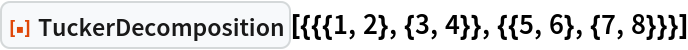Out=Compute the Tucker decomposition with a specified rank:

 In:=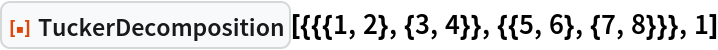Out=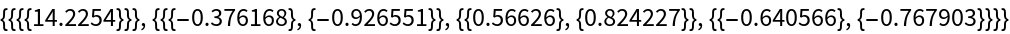### Scope (3)

Specify a list of ranks for each tensor dimension:

 In:=Out=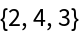For a scalar or a vector (tensors with rank 0 and 1), the Tucker decomposition yields an empty list of factors, and the core is identical to the input tensor itself:

 In:=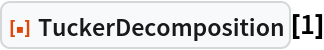Out=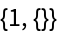In:=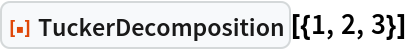Out=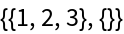Tucker decomposition of a sparse tensor:

 In:=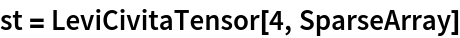Out=In:=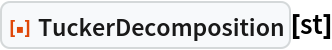Out=### Options (1)

#### Tolerance (1)

Compute the Tucker decomposition with a custom tolerance:

 In:=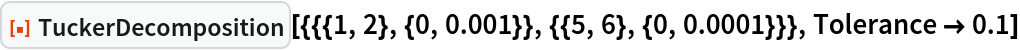Out=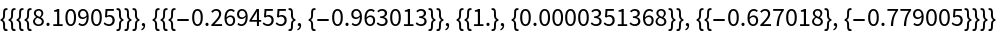### Properties and Relations (2)

Compute the Tucker decomposition of a tensor:

 In:=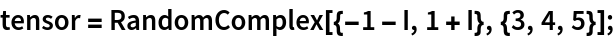In:=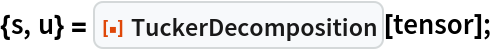The factor matrices are unitary matrices:

 In:=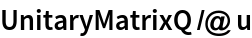Out=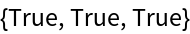To recover the original tensor, one can use the resource function EinsteinSummation to contract corresponding tensor indices:

 In:=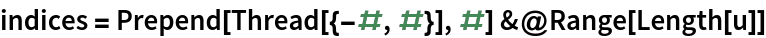Out=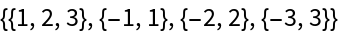In:=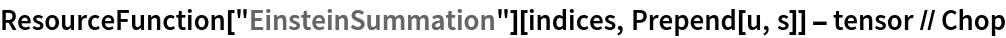Out=For a complex square matrix, TuckerDecomposition returns the core tensor as a matrix, along with the factor matrices:

 In:=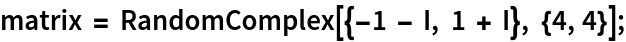In:=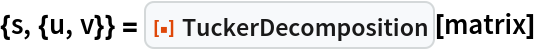Out=The core tensor is not a diagonal matrix:

 In:=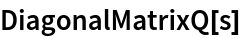Out=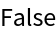Recover the original matrix as u.s.Transpose[v]:

 In:=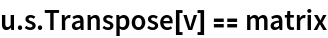Out=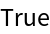SingularValueDecomposition returns a diagonal core matrix s and conjugated factor matrices:

 In:=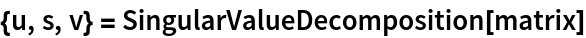Out=In:=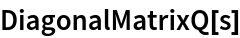Out=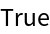In:=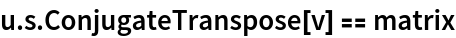Out=## Version History

• 1.0.0 – 10 April 2023